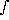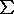# Dobson Units

DOBSON10-3 cm-amagat
given the number density, N, in molecules per cm3 thenx = (<mw>x · Nx(z))/Na grams/cm2
C(L)N(z) dz<N(L)> ·z = <N(i)> ·P/(t g) = q · Na ·P/(<mw> g) molecules/cm2
Note that= q ·t = N · <mw> /Na where q is the volumetric mixing ratio of the species being measured.
To convert to mass column density, M, in grams per cm2
M(L) = (<mw>O3 /Na) · C(L) =P/ g
DOBSON = 1000 * Z = 1000 *C(L) / N0
where N0 is Loschmidt's number.

The atmosphere consists of fixed gases (e.g., CO2, N2O, CO), water, and ozone so the pressure within any level is given asp(L)t g dz = g(f +w +o) dz
so thatp(L) · Na / g = <mw>f · Cf(L) + <mw>w · Cw(L) + <mw>o · Co(L)
Cf(L) = (p(L) · Na)/(<mw>f · g) - (<mw>w/<mw>f) · Cw(L) - (<mw>o/<mw>f) · Co(L)
we can also define the total column density as
Ct(L)(p(L) · Na) / (<mw>(L) · g)
and if we require
Ct(L) = Cf(L) + Cw(L) + Co(L)
then,
<mw>(L) = (<mw>f · Cf(L) + <mw>w · Cw(L) + <mw>o · Co(L)) / Ct(L)
Ct(L) in eqn 6 and <mw> can be solved for iteratively with an initial guess of <mw> = <mw>f
The volumetric mixing ratio, i.e., molecules of species x to the total number of molecules, is given by
fo(L) = Co(L) / Ct(L) = Co(L) / (P(L) · Na) · <mw>(L) · g